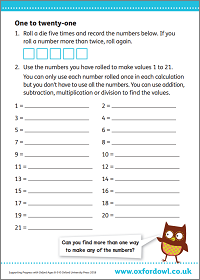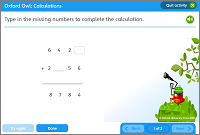We use cookies to enhance your experience on our website. By continuing to use our website, you are agreeing to our use of cookies. You can change your cookie settings at any time. Find out more# Addition & subtraction in Year 5 (age 9–10)

In Year 5, your child will solve problems involving numbers with more than four digits. They will be able to mentally solve problems involving large numbers, and will tackle longer multi-step calculations.

The key words for this section are area/grid methoddifference, and inverse.

## What your child will learn

Take a look at the National Curriculum expectations for addition and subtraction in Year 5 (ages 9–10):

#### Add and subtract whole numbers with more than four digits

Your child will learn to solve addition and subtraction problems involving numbers with more than four digits. They should be able to use lots of different methods, including using objects, diagrams (such as the area/grid method and number lines), and written methods like column addition and column subtraction.

#### Add and subtract numbers mentally with increasingly large numbers

Your child will practise a range of mental calculation strategies to solve addition and subtraction problems. They will be expected to explain how they have solved problems mentally.

#### Use rounding to check answers and make estimates

Your child will use rounding to estimate answers. They will then need to check that their answers are sensible and accurate.

In Year 5, they will also need to start making judgments about what level of accuracy to round to in the context of a problem. For example, if adding 1,046,462 and 6,852,468, it might make sense to round to the nearest 1000, but when adding £3.15 and £2.74, it might make sense to round to the nearest pound or 10p.

#### Solve multi-step problems in contexts

In Year 5, your child will be expected to solve multi-step word problems. These are word-based maths problems that require your child to solve multiple calculations before coming to the answer.

The multi-step word problem could involve any combination of up to three operations (addition, subtraction, multiplication, and division). Your child should have a range of methods to solve these problems.

Your child will need to explain what method they used to solve a problem and to explain why they used that method. They also need to be able to check their answer using a different method.

## How to help at home

There are lots of quick and easy ways you can help your child to understand addition and subtraction. Here are just a couple of ideas to support your child’s learning:

### 1. Play the Number Card game

1. Make two sets of number cards from 0–9.
2. Put the cards face down and choose 4 cards each.
3. Each player makes their 4 cards into a four-digit number and tells their partner what number they have made.
4. Flip a coin to decide whether you are going to add or subtract the two four-digit numbers (heads = add, tails = subtract). If you are subtracting, discuss which number you will subtract from which.
5. Each player solves the addition or subtraction problem.
6. Players check they have arrived at the same answer. If not, work out where the error was made.

Compare the strategies you both used to solve the problem. Try using each other’s strategy and/or find as many different ways you can to solve the problem. This game can be entirely cooperative, or you could introduce a hint of competition by seeing who can solve the problem first!

Try these activities for more addition and subtraction practice:

### Activity: One to twenty-oneFind ways to add up to all the numbers 0–21.Fill in the missing numbers in these addition and subtraction calculations.

## 2. Try the rounding challenge

Rounding is an important skill your child will be expected to use to estimate and to check answers to calculations.

You could help your child to practise rounding with the calculations below. For each sum, you and your child could round the numbers to the nearest 10, 100, or 1000 in order to estimate the answer. Which one of you can get the estimate fastest? Then ask your child to work out the correct answer. Whose estimate was closest to the real answer? You can add an extra element of challenge by using a stopwatch or timer!

3567 + 2332

6102 + 2923

1345 + 3348

8799 – 4889

9833 – 5541